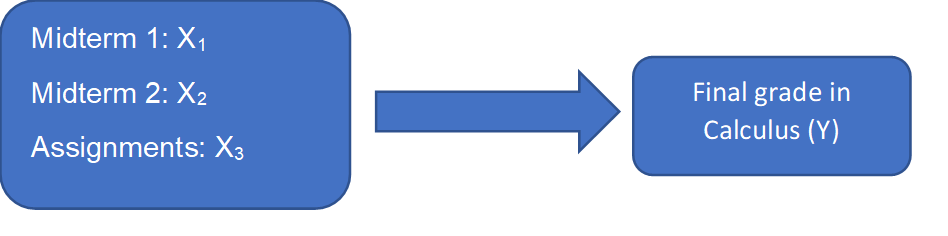Search

# Multiple Linear Regression – What and Why?

Even though Linear regression is a useful tool, it has significant limitations. It can only be fit to datasets that has one independent variable and one dependent variable.

When we have data set with many variables, Multiple Linear Regression comes handy. While it can’t address all the limitations of Linear regression, it is specifically designed to develop regressions models with one dependent variable and multiple independent variables or vice versa.

The different variations in Multiple Linear Regression model are:

1. Multiple Regression – One dependent variable (Y), more than one Independent

variables(X)

2. MultiVariate Regression - more than one dependent variables(Y), One independent

variable (X)

3. MultiVariate Multiple Regression – more than 1 dependent (Y) and Independent (X)

variables.

The most widely used one is Multiple regression model.

What is Multiple Linear Regression?

Multiple regression is a statistical method that aims to predict a dependent variable using multiple independent variables. It is generally used to find the relationship between several independent variables and a dependent variable.

The formula for Multiple regression model is:

Y = b1*X1 + b2*X2 + … + bn*Xn + A

Where, Y denotes the predicted value

b1, b2, … bn are the regression coefficients, which represent the value at which

the X variable changes when the Y variable changes.

X1, X2, … Xn are independent variables

A is the Y intercept

Example

The following example demonstrates an application of multiple regression to a real-life situation:

A high school student has concerns over his coming final Math Calculus exam. In response, his teacher outlines how he can estimate his final grade on the subject through consideration of the grades he received throughout the school year.

Take a look at the diagrammatic representation of all variables in this example:The student can predict his final exam grade (Y) using the three scores identified above (X1, X2, X3).

We can now use the prediction equation to estimate his final exam grade. Assuming the regression coefficients for Midterm 1(X1) as 0.38, Midterm 2(X2) as 0.42 and Assignment grades(X3) as 0.61 and Y intercept(A) as -5.70 results in the following equation:

ŷ = -5.70 + 0.38*Term1 + 0.42*Term2 + 0.61*Assign

Under the assumption that the student scored 70% on Term 1, 60% on term 2 and 80% on the assignments, his predicted final exam grade would have been:

ŷ = -5.70 + 0.38*(70) + 0.42*(60) + 0.16*(80)

ŷ = 58.9

So, the student might expect to receive a 58.9 on his Calculus final exam.

Hope I was able to explain multiple regression in a simple and understandable way.

Key Takeaways:

These are some major uses for multiple linear regression analysis.

1. It can be used to forecast effects or impacts of changes. That is, multiple linear regression analysis helps us to understand how much the dependent variable will change when we change the independent variables.

2. Multiple linear regression analysis predicts trends and future values. The multiple linear regression analysis can be used to get point estimates. An example question might be “what will the price of gold be in 6 months from now?”